# Absolute value facts for kids

Kids Encyclopedia Facts

In mathematics, the absolute value or modulus of a real number is the number without the sign. The absolute value of 2 is 2, the absolute value of -2 is also 2. This notation is to express a number's distance from zero on a number line. The absolute value of 10 would be 10 since the number 10 is 10 numbers away from zero, same follows with negatives.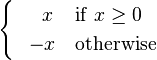$\begin{cases} \ \;\,\ \ x &\mathrm{if}\ x \ge 0\\ \ \;\, - x &\mathrm{otherwise} \end{cases}$

The modulus of a complex number is given by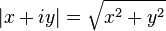$|x + iy| = \sqrt{x^2+y^2}\$

## Properties

### Real numbers

For any real number x the absolute value of x is denoted by | x | (a vertical bar on each side of the quantity) and is defined as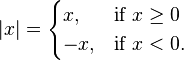$|x| = \begin{cases} x, & \mbox{if } x \ge 0 \\ -x, & \mbox{if } x < 0. \end{cases}$

The absolute value of x is always either positive or zero, but never negative. From an analytic geometry point of view, the absolute value of a real number is that number's distance from zero along the real number line. The absolute value of the difference of two real numbers is the distance between them.

In calculus, the absolute value function is differentiable except at 0. It is everywhere continuous.

Compare the norm of a vector.

The square-root notation without sign represents the positive square root. So, it follows that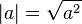$|a| = \sqrt{a^2}$ (1)

which is sometimes used as a definition of absolute value.

The absolute value has the following four main properties: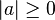$|a| \ge 0$ (2) Non-negativity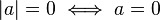$|a| = 0 \iff a = 0$ (3) Positive-definiteness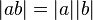$|ab| = |a||b|\,$ (4) Multiplicativeness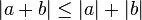$|a+b| \le |a| + |b|$ (5) Subadditivity

Other important properties of the absolute value include: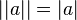$||a|| = |a|\,$ (6) Idempotence (the absolute value of the absolute value is the absolute value)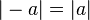$|-a| = |a|\,$ (7) Symmetry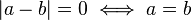$|a - b| = 0 \iff a = b$ (8) Identity of indiscernibles (equivalent to positive-definiteness)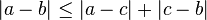$|a - b| \le |a - c| +|c - b|$ (9) Triangle inequality (equivalent to subadditivity)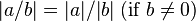$|a/b| = |a| / |b| \mbox{ (if } b \ne 0) \,$ (10) Preservation of division (equivalent to multiplicativeness)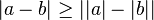$|a-b| \ge ||a| - |b||$ (11) (equivalent to subadditivity)

Two other useful properties concerning inequalities are: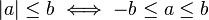$|a| \le b \iff -b \le a \le b$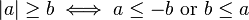$|a| \ge b \iff a \le -b \mbox{ or } b \le a$

These relations may be used to solve inequalities involving absolute values. For example: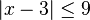$|x-3| \le 9$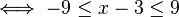$\iff -9 \le x-3 \le 9$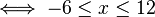$\iff -6 \le x \le 12$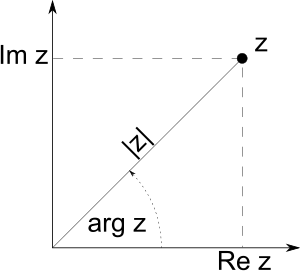Diagram showing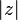$|z|$, the modulus of z.

### Complex numbers

For a complex number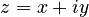$z = x + iy$, where x is the real part of z and iy is the imaginary part of z,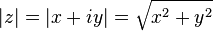$|z| = |x + iy| = \sqrt{x^2+y^2}\$

## Images for kidsAbsolute value Facts for Kids. Kiddle Encyclopedia.